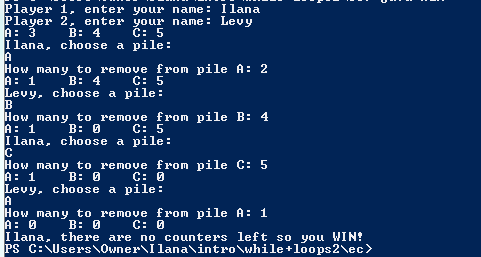# Project 2

```///Name: Ilana Levy
///Period: 5
///Program Name: Nim
///File Name: nim.java
///Date Finished: 10/10/2015

import java.util.Scanner;

public class nim
{
public static void main( String[] args )
{
Scanner keyboard = new Scanner( System.in );
String player1, player2, pile;
int remove, A = 3, B = 4, C = 5, D = A + B + C;

System.out.print( "Player 1, enter your name: " );
player1 = keyboard.next();
System.out.print( "Player 2, enter your name: " );
player2 = keyboard.next();

while( (A + B + C ) > 0 )
{

System.out.println( "A: " + A + "\tB: " + B + "\tC: " + C + "\n" + player1 + ", choose a pile: " );

pile = keyboard.next();

if (pile.equals( "A" ) && A > 0)
{
System.out.print( "How many to remove from pile " + pile + ": " );
remove = keyboard.nextInt();

A = A - remove;
}
else if ( pile.equals( "B" ) && B > 0)
{
System.out.print( "How many to remove from pile " + pile + ": " );
remove = keyboard.nextInt();

B = B - remove;
}
else if ( pile.equals( "C" ) && C > 0 )
{
System.out.print( "How many to remove from pile " + pile + ": " );
remove = keyboard.nextInt();

C = C - remove;
}

if (A + B + C > 0 )
{
System.out.println( "A: " + A + "\tB: " + B + "\tC: " + C + "\n" + player2 + ", choose a pile: " );

pile = keyboard.next();
if (pile.equals( "A" ) )
{

System.out.print( "How many to remove from pile " + pile + ": " );
remove = keyboard.nextInt();

A = A - remove;
}
else if ( pile.equals( "B" ) )
{
System.out.print( "How many to remove from pile " + pile + ": " );
remove = keyboard.nextInt();

B = B - remove;
}
else if ( pile.equals( "C" ) )
{
System.out.print( "How many to remove from pile " + pile + ": " );
remove = keyboard.nextInt();

C = C - remove;
}

}
}

System.out.println( "A: " + A + "\tB: " + B + "\tC: " + C + "\n" + player1 + ", there are no counters left so you WIN! " );

}

}
```